# RD Sharma Solutions Class 9 Maths Chapter 24 Measures Of Central Tendency Exercise 24.2

Class 9 Chapter 24 – Measure of Central Tendency Exercise 24.2 solutions are provided here. RD Sharma solutions for class 9 – Measures of Central Tendency is the best tool to prepare for your exams. This will help to guide you through the questions you have trouble while trying to solve RD Sharma for class 9. These solutions for class 9 textbook are outlined and solved by the experts at BYJU’S.

## Download PDF of RD Sharma Solutions for Class 9 Maths Chapter 24 Measure of Central Tendency Exercise 24.2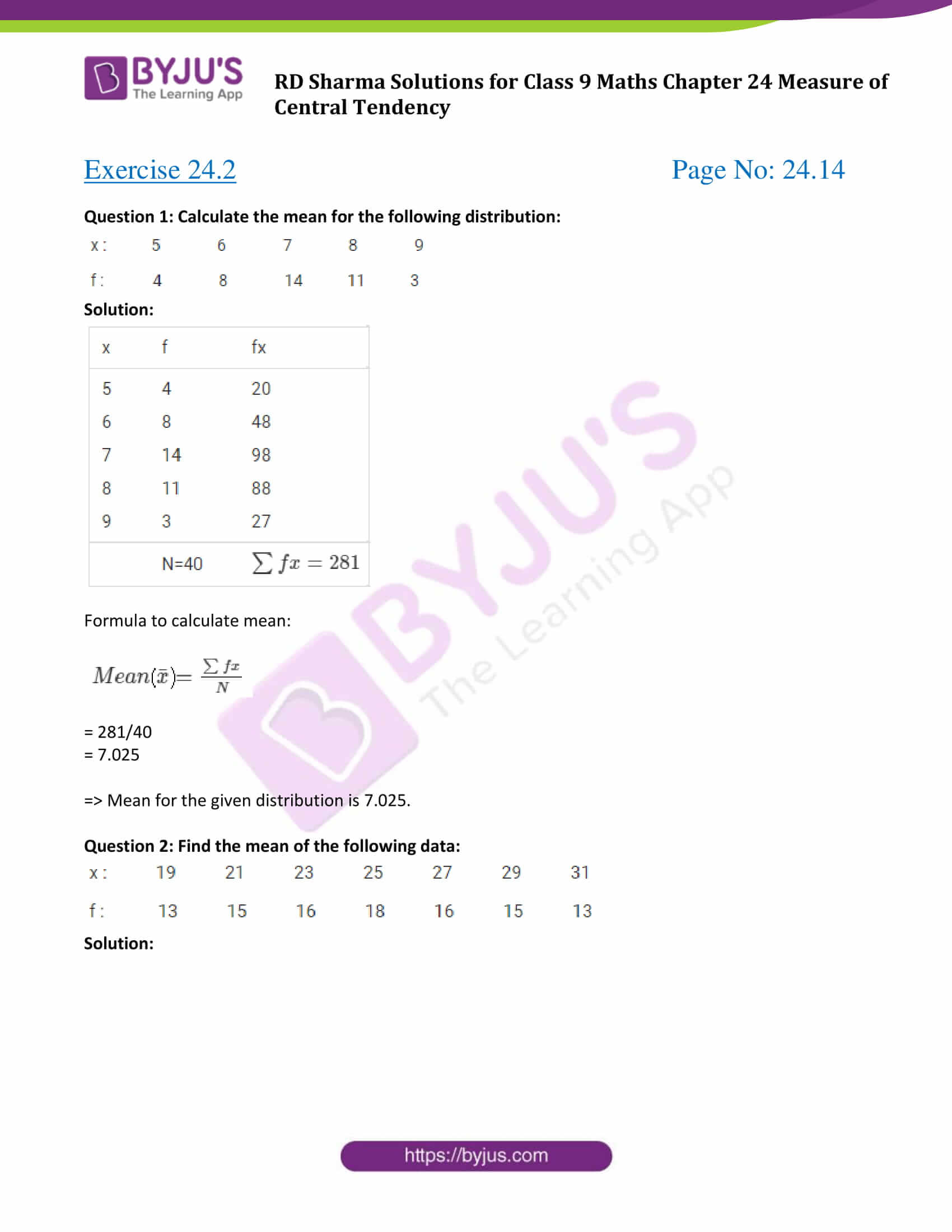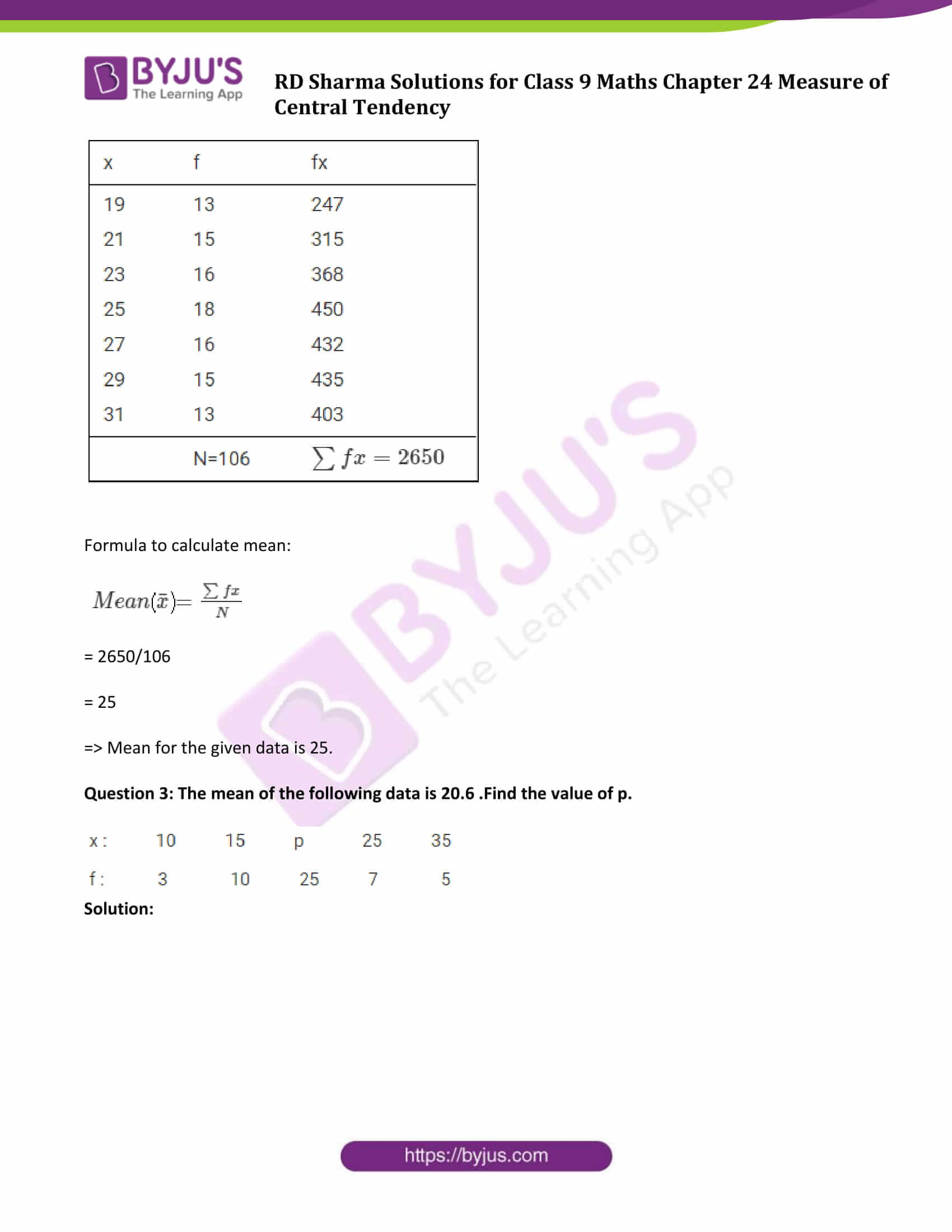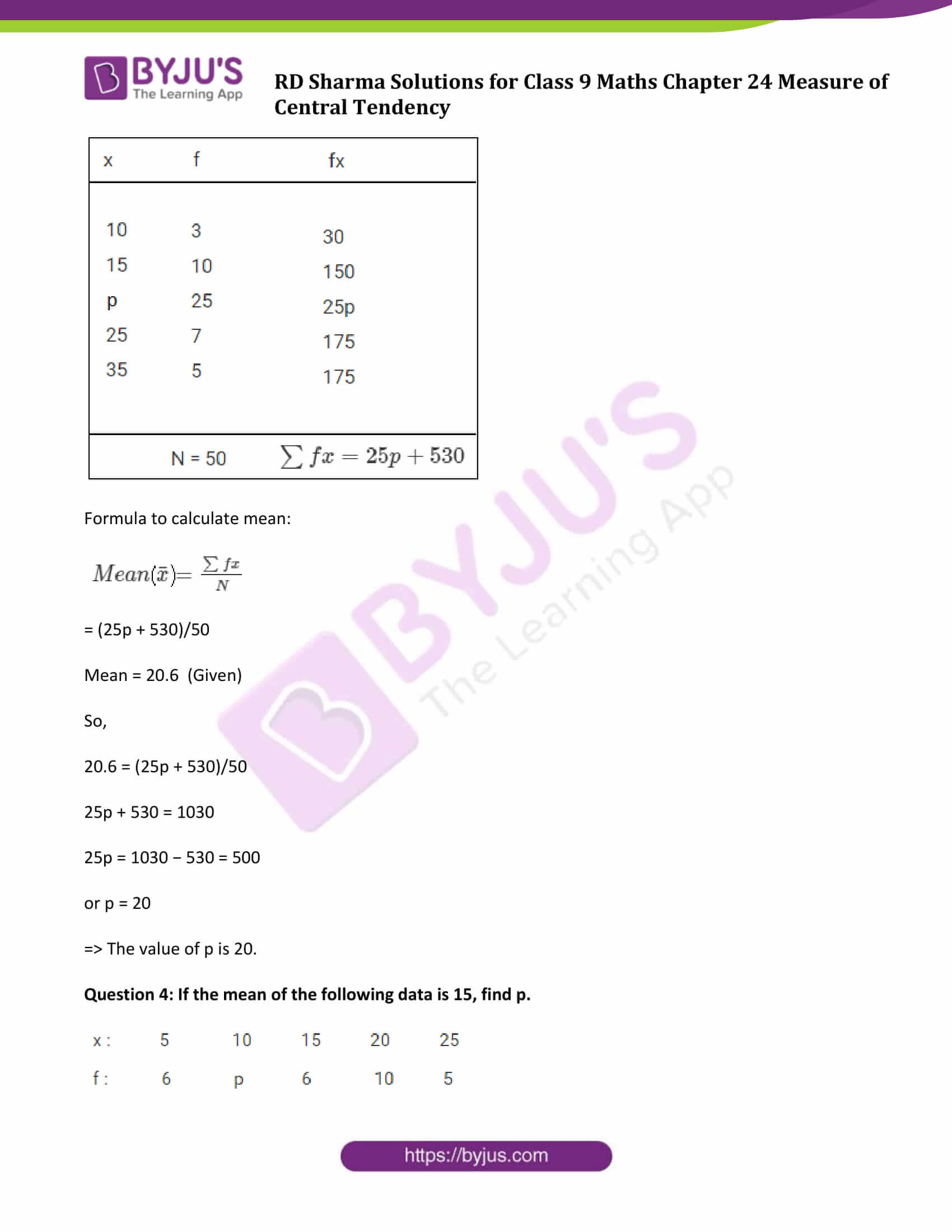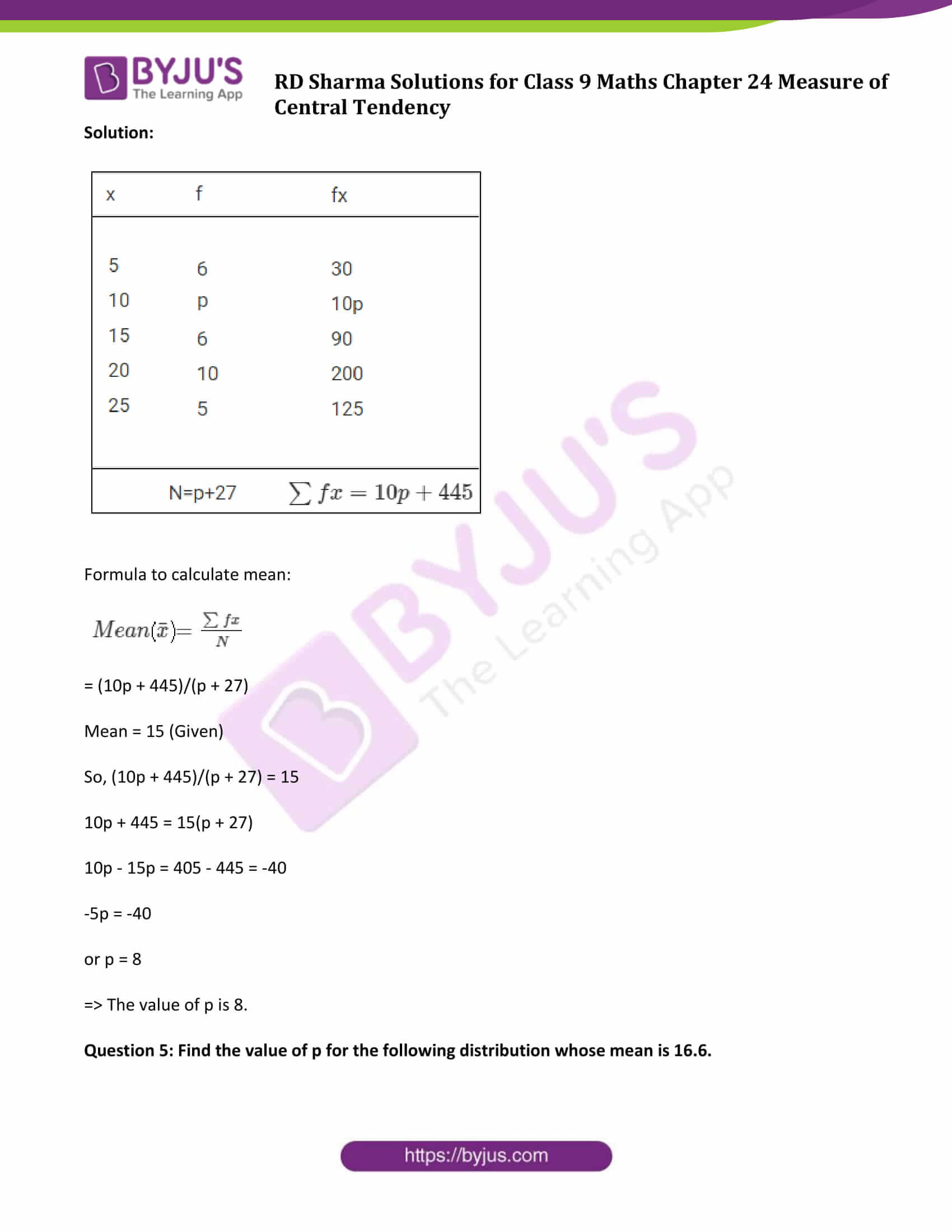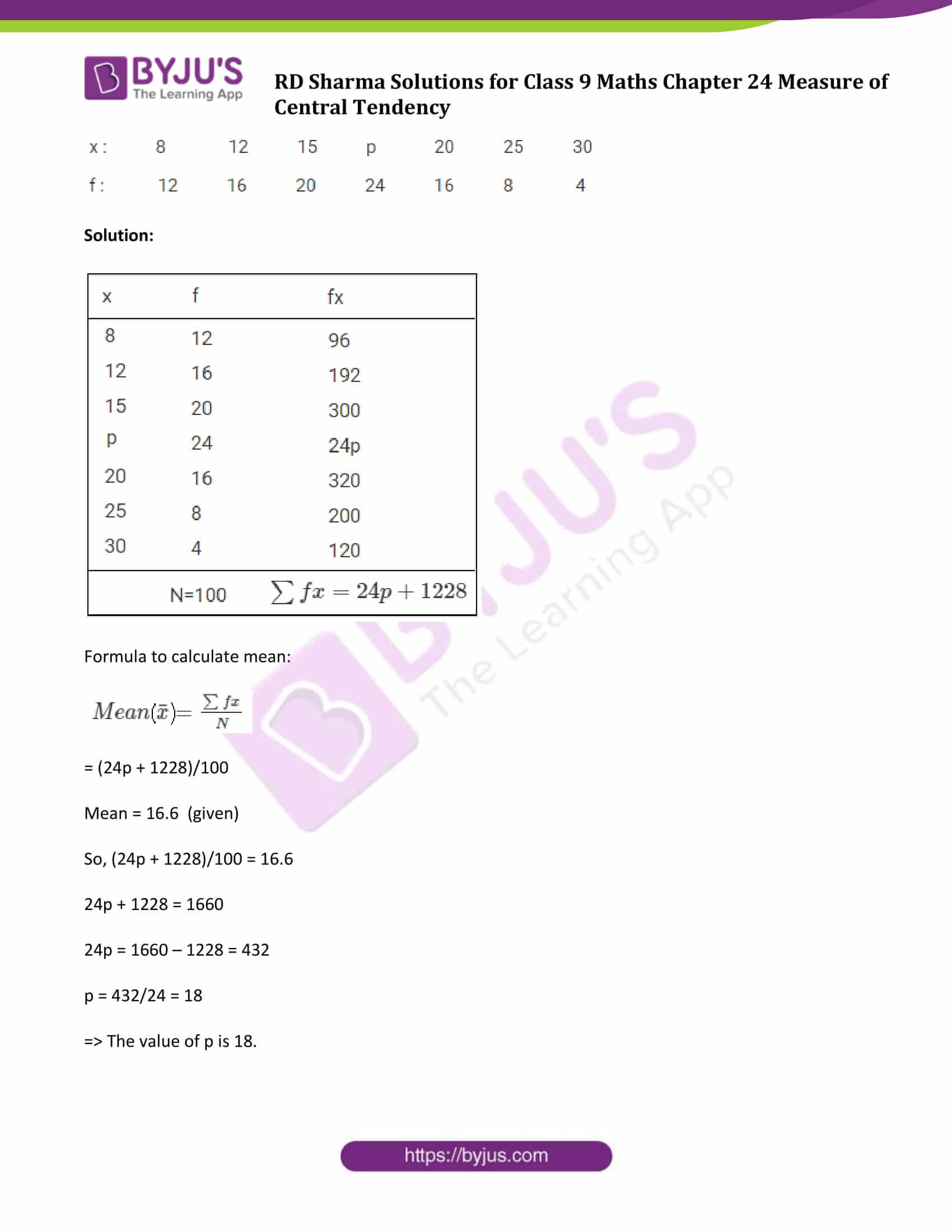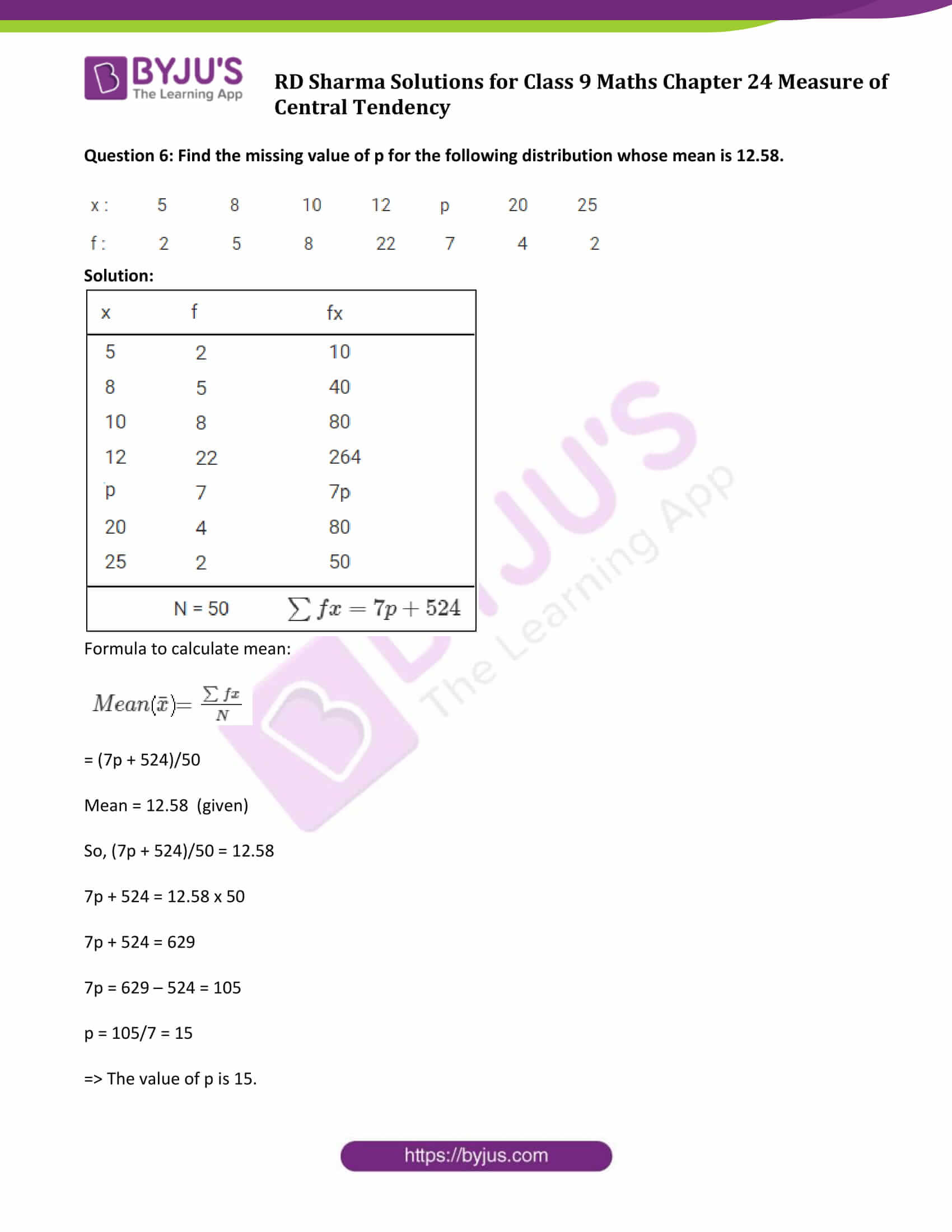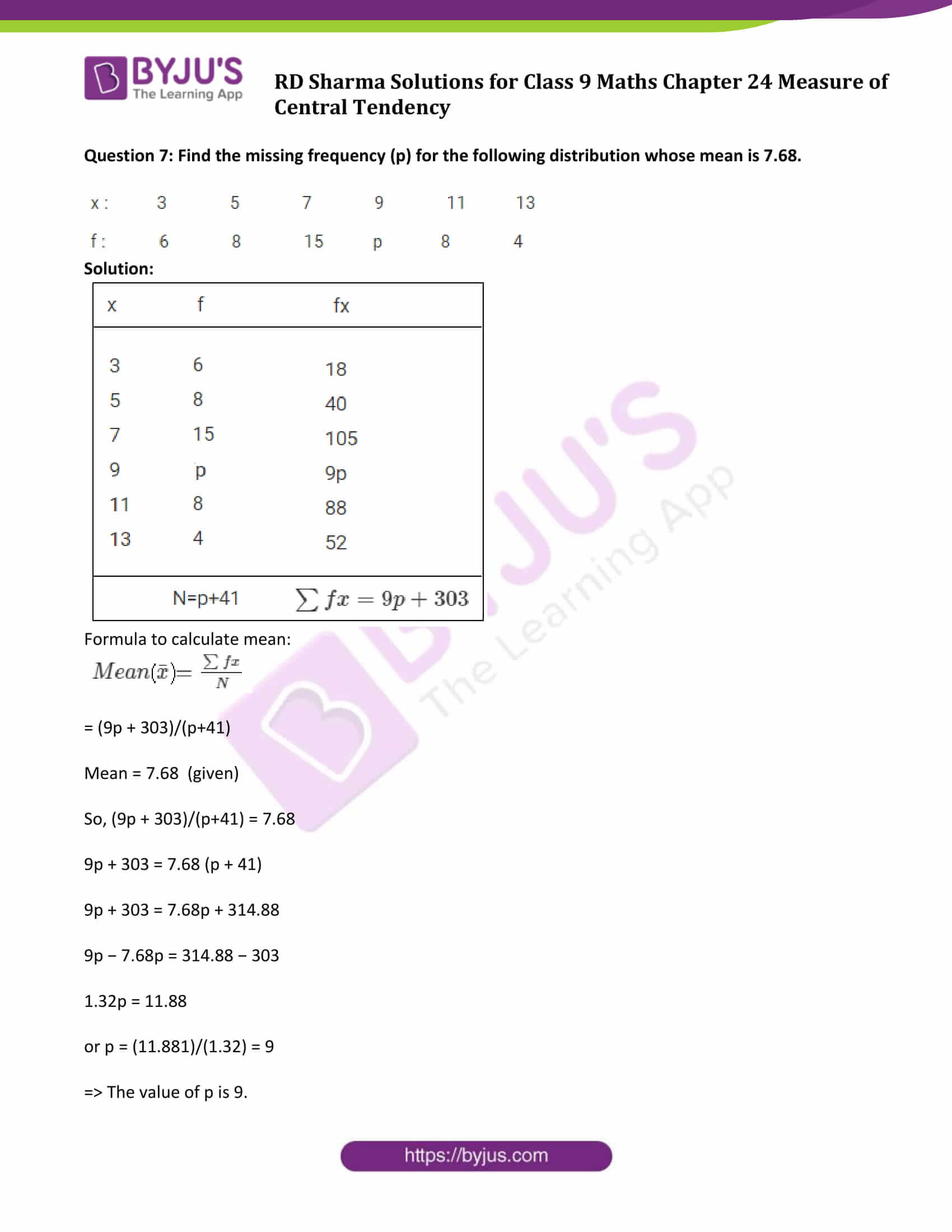### Access Answers to Maths RD Sharma Solutions for Class 9 Chapter 24 Measure of Central Tendency Exercise 24.2 Page number 24.14

Question 1: Calculate the mean for the following distribution: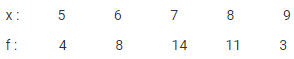Solution: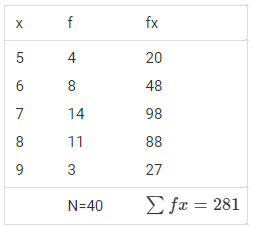Formula to calculate mean: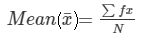= 281/40

= 7.025

⇒ Mean for the given distribution is 7.025.

Question 2: Find the mean of the following data: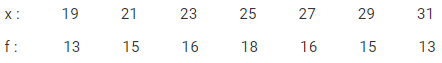Solution: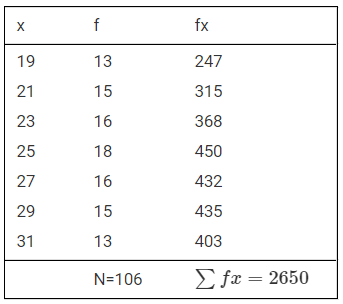Formula to calculate mean:= 2650/106

= 25

⇒ Mean for the given data is 25.

Question 3: The mean of the following data is 20.6 .Find the value of p.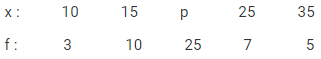Solution: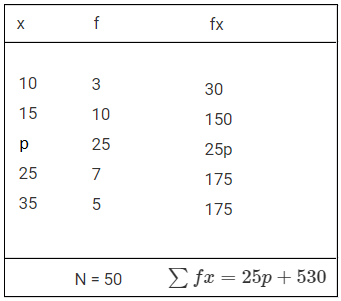Formula to calculate mean:= (25p + 530)/50

Mean = 20.6 (Given)

So,

20.6 = (25p + 530)/50

25p + 530 = 1030

25p = 1030 − 530 = 500

or p = 20

⇒ The value of p is 20.

Question 4: If the mean of the following data is 15, find p.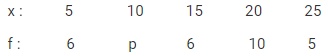Solution: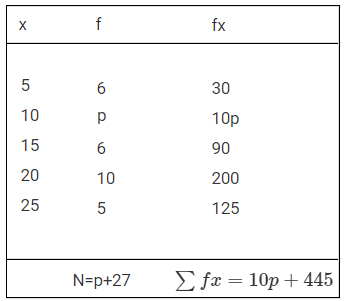Formula to calculate mean:= (10p + 445)/(p + 27)

Mean = 15 (Given)

So, (10p + 445)/(p + 27) = 15

10p + 445 = 15(p + 27)

10p – 15p = 405 – 445 = -40

-5p = -40

or p = 8

⇒ The value of p is 8.

Question 5: Find the value of p for the following distribution whose mean is 16.6.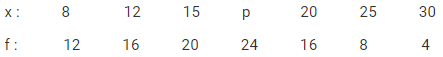Solution: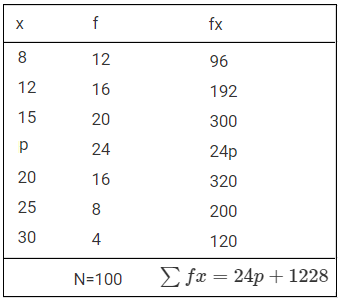Formula to calculate mean:= (24p + 1228)/100

Mean = 16.6 (given)

So, (24p + 1228)/100 = 16.6

24p + 1228 = 1660

24p = 1660 – 1228 = 432

p = 432/24 = 18

⇒ The value of p is 18.

Question 6: Find the missing value of p for the following distribution whose mean is 12.58.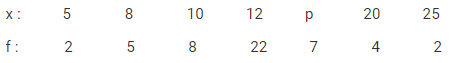Solution: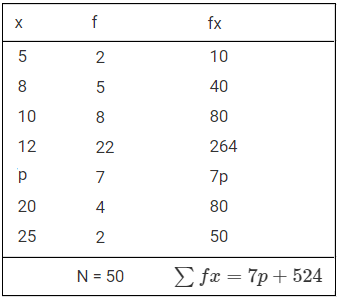Formula to calculate mean:= (7p + 524)/50

Mean = 12.58 (given)

So, (7p + 524)/50 = 12.58

7p + 524 = 12.58 x 50

7p + 524 = 629

7p = 629 – 524 = 105

p = 105/7 = 15

⇒ The value of p is 15.

Question 7: Find the missing frequency (p) for the following distribution whose mean is 7.68.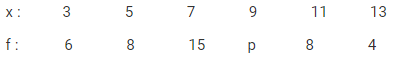Solution: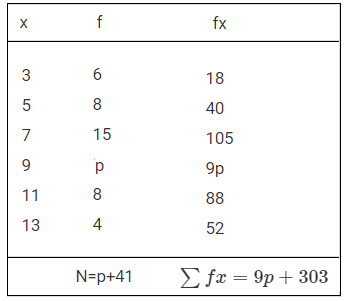Formula to calculate mean:= (9p + 303)/(p+41)

Mean = 7.68 (given)

So, (9p + 303)/(p+41) = 7.68

9p + 303 = 7.68 (p + 41)

9p + 303 = 7.68p + 314.88

9p − 7.68p = 314.88 − 303

1.32p = 11.88

or p = (11.881)/(1.32) = 9

⇒ The value of p is 9.

## RD Sharma Solutions for Class 9 Maths Chapter 24 Measures of Central Tendency Exercise 24.2

RD Sharma Solutions Class 9 Maths Chapter 24 Measures of Central Tendency Exercise 24.2 is based on the following topics:

• Arithmetic mean of grouped data or discrete frequency distribution
• Methods to solve Arithmetic mean – Direct Method, Short-cut Method, step deviation method